# 随机梯度下降法

44 篇文章 1 订阅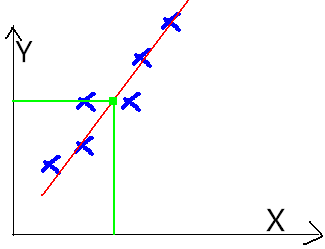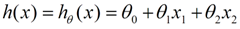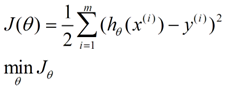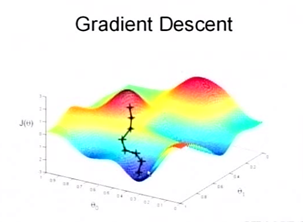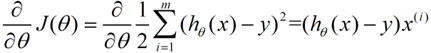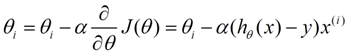（α表示算法的学习速率）

• 梯度下降：梯度下降就是我上面的推导，要留意，在梯度下降中，对于 θ θ 的更新，所有的样本都有贡献，也就是参与调整 θ θ .其计算得到的是一个标准梯度。因而理论上来说一次更新的幅度是比较大的。如果样本不多的情况下，当然是这样收敛的速度会更快啦~

• 随机梯度下降：可以看到多了随机两个字，随机也就是说我用样本中的一个例子来近似我所有的样本，来调整 θ θ ，因而随机梯度下降是会带来一定的问题，因为计算得到的并不是准确的一个梯度，容易陷入到局部最优解中

• 批量梯度下降：其实批量的梯度下降就是一种折中的方法，他用了一些小样本来近似全部的，其本质就是我1个指不定不太准，那我用个30个50个样本那比随机的要准不少了吧，而且批量的话还是非常可以反映样本的一个分布情况的。

#Python 3.3.5
import random
# matrix_A  训练集
matrix_A = [[1,4], [2,5], [5,1], [4,2]]
Matrix_y = [19,26,19,20]
theta = [2,5]
#学习速率
leraing_rate = 0.005
loss = 50
iters = 1
Eps = 0.0001
#随机梯度下降
while loss>Eps and iters <1000 :
loss = 0
i = random.randint(0, 3)
h = theta*matrix_A[i] + theta*matrix_A[i]
theta = theta + leraing_rate*(Matrix_y[i]-h)*matrix_A[i]
theta = theta + leraing_rate*(Matrix_y[i]-h)*matrix_A[i]
Error = 0
Error = theta*matrix_A[i] + theta*matrix_A[i] - Matrix_y[i]
Error = Error*Error
loss = loss +Error
iters = iters +1
print ('theta=',theta)
print ('iters=',iters)
"""
#梯度下降
while loss>Eps and iters <1000 :
loss = 0
for i in range(4):
h = theta*matrix_A[i] + theta*matrix_A[i]
theta = theta + leraing_rate*(Matrix_y[i]-h)*matrix_A[i]
theta = theta + leraing_rate*(Matrix_y[i]-h)*matrix_A[i]
for i in range(4):
Error = 0
Error = theta*matrix_A[i] + theta*matrix_A[i] - Matrix_y[i]
Error = Error*Error
loss = loss +Error
iters = iters +1
print ('theta=',theta)
print ('iters=',iters)
"""
"""
#批量梯度下降
while loss>Eps and iters <1000 :
loss = 0
sampleindex =  random.sample([0,1,2,3],2)
for i in sampleindex :
h = theta*matrix_A[i] + theta*matrix_A[i]
theta = theta + leraing_rate*(Matrix_y[i]-h)*matrix_A[i]
theta = theta + leraing_rate*(Matrix_y[i]-h)*matrix_A[i]
for i in sampleindex :
Error = 0
Error = theta*matrix_A[i] + theta*matrix_A[i] - Matrix_y[i]
Error = Error*Error
loss = loss +Error
iters = iters +1
print ('theta=',theta)
print ('iters=',iters)
"""

>>>
theta= [2.9980959216157945, 4.001522800837675]
iters= 75

matrix_A = [[1.05,4], [2.1,5], [5,1], [4,2]]

>>>
theta= [3.0095950685197725, 3.944718521027671]
iters= 1000

07-1206-272314
06-161万+
01-042万+
03-082184
09-077636
11-215562
10-012万+
11-033万+
10-21185
11-28893
09-20
06-087329
05-166784
10-074368点击重新获取扫码支付余额充值